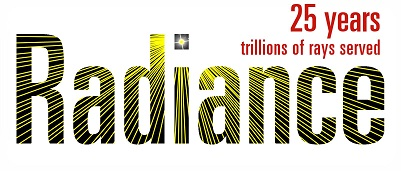# Ambient divisions

Hi Everyone

I have some problems specifying the amount of ambient divisions I want to use. When using rtrace with -otv the amount of rays I’m seeing is usually less than what I have specified.

I thought that maybe interpolated values are not reported so I resorted to aa=0, which reduced the amount of rays even further.

So, how is the number of divisions actually controlled and determined?

Thanks a lot!

The number of ambient divisions is rounded down the the nearest perfect square below the number specified. At deeper levels of the ray tree, the number of divisions (and super-samples) is further reduced by 0.5^N, where N is the number of bounces. This is all in the name of saving caclulation time while maintaining a constant contribution to accuracy. The 0.5 value was selected as a compromise average reflectance value, which is higher than it should be in some cases and lower than it should be in others.

If you set -aa 0, then the actual surface reflectance or transmittance is used rather than 0.5, since the values are not cached or re-used.

Thanks Greg!

So, according to this I should expect the number of reported rays to change with reflectivity?

I’ve schemed through the code, and the closes thing I could find is this part, in samp_hemi under ambient.c:

``````if (ambacc <= FTINY &&
wt > (d = 0.8*intens(rcol)*r->rweight/(ambdiv*minweight)))
wt = d;			/* avoid ray termination */
n = sqrt(ambdiv * wt) + 0.5;
i = 1 + 5*(ambacc > FTINY);	/* minimum number of samples */
``````

However I can’t seem to correlate this with your description, perhaps I’m looking at the wrong place?

Thanks

Very good. You are in the right place, but as usual, the logic is a bit convoluted. The sqrt() function assigned to an integer gets the nearest perfect square, as n*n is used later as the number of samples. The “wt” is passed either 0.5^N or the product of surface reflectances, depending on whether -aa is 0 or not.

Just to add, this relaxation of ad rays works extremely well for most realistic scenarios because, with increasing number of bounces, the (diffuse) illumination field tends towards becoming isotropic. If you want to see a practical example showing how this affects the number of sampling points from ab 1 to ab 7, see ``Section 3.3.3 Ambient calculation - progression and convergence characteristics’’ (p89):

https://repository.lboro.ac.uk/articles/Daylight_simulation_validation_sky_models_and_daylight_coefficients/9460817

(Apologies, it’s buried deep in a 20mb PDF.)

I noticed something interesting:

in this line n = sqrt(ambdiv * wt) + 0.5;

Assuming aa=0, wt is the reflectance divided by the ambient divisions. I deduce this from line 274-277 of the function “samp_hemi” in ambocomp.c:

``````copycolor(hp->acoef, rcol);
d = 1.0/(n*n);
scalecolor(hp->acoef, d);
``````

However, this means that as we reach recursion level 2, ambdivision*wt
cannot be larger than 1, and so we end up with 1 division ray.

Later recursion levels will also have just 1 ray since since wt does not increase.

Indeed what I’m seeing is that when aa=0, past the first recursion level I never get more than 1 ambient divisions. Am I missing something, or is this the intended behaviour?

Well, it depends somewhat on what -ad starts out with and your surface reflectances, but yes, the intended behavior is to send a single ray per level past a couple of bounces, mimicking path tracing when -aa is set to 0.